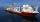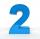Prism bases

Volume perpendicular quadrilateral prism is 360 cm3. The edges of the base and height of the prism are in the ratio 5:4:2 Determine the area of the base and walls of the prism.

Result

S1 =  86.535 cm2
S2 =  34.614 cm2
S3 =  43.267 cm2

Solution:Leave us a comment of example and its solution (i.e. if it is still somewhat unclear...):Be the first to comment!To solve this verbal math problem are needed these knowledge from mathematics:

Tip: Our volume units converter will help you with converion of volume units.

Next similar examples:

1. Cuboid edges in ratioCuboid edges lengths are in ratio 2:4:6. Calculate their lengths if you know that the cuboid volume is 24576 cm3.
2. Scale factorA prism with a volume of 1458 mm3 is scaled down to a volume of 16mm3. What is the scale factor in fraction form?
3. Truncated coneCalculate the height of the rotating truncated cone with volume V = 1115 cm3 and a base radii r1 = 7.9 cm and r2 = 9.7 cm.
4. Two boxes-cubesTwo boxes cube with edges a=38 cm and b = 81 cm is to be replaced by one cube-shaped box (same overall volume). How long will be its edge?
5. Cube cornersFrom cube of edge 14 cm cut off all vertices so that each cutting plane intersects the edges 1 cm from the nearest vertice. How many edges will have this body?
6. Cross-sections of a coneCone with base radius 16 cm and height 11 cm divide by parallel planes to base into three bodies. The planes divide the height of the cone into three equal parts. Determine the volume ratio of the maximum and minimum of the resulting body.
7. TetrahedronCalculate height and volume of a regular tetrahedron whose edge has a length 18 cm.
8. The volume 2The volume of a cube is 27 cubic meters. Find the height of the cube.
9. Gas consumptionThe vessel consume 100 tons of gas in 250 miles. How many fuel will the vessel consume if it travels 400 miles?
10. BottlesThe must is sold in 5-liter and 2-liter bottles. Mr Kucera bought a total of 216 liters in 60 bottles. How many liters did Mr. Kucera buy in five-liter bottles?
11. The bulbsThe bulbs are connected serially two bulbs and one resistor. Each bulb has a resistivity of 100 ohms and resistor of 60 ohms The voltage source in the circuit is 24 volts. Do you know what voltage to measure on individual appliances?
12. Holidays - on poolChildren's tickets to the swimming pool stands x € for an adult is € 2 more expensive. There was m children in the swimming pool and adults three times less. How many euros make treasurer for pool entry?
13. Theorem proveWe want to prove the sentence: If the natural number n is divisible by six, then n is divisible by three. From what assumption we started?
14. Numbers at ratioThe two numbers are in a ratio 3:2. If we each increase by 5 would be at a ratio of 4:3. What is the sum of original numbers?
15. AlgebraX+y=5, find xy (find the product of x and y if x+y = 5)
16. CoefficientDetermine the coefficient of this sequence: 7.2; 2.4; 0.8
17. Expression with powersIf x-1/x=5, find the value of x4+1/x4